ave## ave

بازدید : 667 پنجشنبه 11 دي 1393

## ()ave

`ave(expr, dim1, dim2 <,tinc> <,-b>)`

Averages the result of `expr` over the specified dimension range. If the averaging dimension is time, an optional time increment `tincr` may be specified.

`expr    `- any valid grads expression
``` dim1    - the start point for the average ``` ``` dim2    - the end point for the average ``` ``` tinc    - optional increment for time averaging ``` ``` -b      - use exact boundaries ```

`dim1` and `dim2` are standard grads dimension expressions whose dimensions must match.

### Usage Notes

1. The limits and intervals of the averaging are set according to the grid coordinates of the default file. If `dim1` and `dim2` are specified in world coordinates, the coordinates are converted to the nearest integer grid coordinates based on the scaling of the default file. See the examples below for further illustration.

2. The end points are given normal weighting, unless the `-b` boundary flag is specified. The boundry flag indicates that the average should be taken to the exact boundaries specified by `dim1` and `dim2`, rather than the nearest grid points.

3. The average is weighted by grid interval to account for non-linear grid spacing. Averages in the latitude dimension are weighted by the difference between the sines of the latitude at the northern and southern edges of the grid box. The edges of the grid box are always defined as being the midpoint between adjacent grid points. To calculate an average without using the latitude weighting, use the `mean` function.

### Examples

For the following examples, the dimension environment is X-Y varying; Z-T are fixed.

1. Consider the following average, when the default file is file #1:

ave(z.2,t=1,t=10)

We are averaging a variable from file #2, but using the scaling from file #1. File #1 has a time interval of 6 hours, but file #2 has a time interval of 12 hours. The average will thus attempt to access data from file #2 for times that are not available, and an error will ocurr. To avoid this, the default file should be set to file #2: `set dfile 2`

2. The average:

ave(z,t=1,t=120,4)

will average only 00Z reports from file #1, since the time increment is 4, which for this file is 24 hours.

3. If you attempt to take a zonal average as follows:

ave(z,lon=0,lon=360)

the world coordinates will be converted to grid coordinates, here `X` varying from 1 to 181, and the grid point at longitude 0 (and 360) will be used twice in the average. To have the end points of this average weighted properly, use the `-b` flag:

ave(z,lon=0,lon=360,-b)

or average using the grid coordinates directly:

ave(z,x=1,x=180)

4. You can nest averaging operations:

ave(ave(z,x=1,x=180),y=1,y=46)

In this case, to take an areal average. Note that for areal averaging, the `aave` function is better. See the `aave` function description.

When nesting averages, the order of the nesting can have a dramatic affect on performance. Keep in mind the ordering of the data in a grads file: X varies the fastest, then Y, then Z, then T. When nesting averages, put the faster varying dimension within the inner average:

`set lon -90 `
`set lat -90 90 `
`set lev 1000 100 `
d ave(ave(t,x=1,x=180),t=1,t=20)

This average would be more efficient than, for example:

ave(ave(t,t=1,t=20),x=1,x=180)

although the final numerical result would be the same.

5. The use of the `define` command can make certain operations much more efficient. If you want to calculate standard deviation, for example:

sqrt(ave(pow(ave(z,t=1,t=20)-z,2),t=1,t=20))

would be correct, but the inside average would be calculated 20 times. Defining the inside average in advance will be substantially faster:

`define zave = ave(z,t=1,t=20) `
`d sqrt(ave(pow(zave-z,2),t=1,t=20)) `
مطالب مرتبط
ارسال نظر برای این مطلب

نام
ایمیل (منتشر نمی‌شود) (لازم)
وبسایت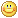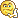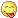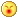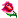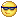نظر خصوصی
مشخصات شما ذخیره شود ؟ [حذف مشخصات] [شکلک ها]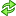کد امنیتی
اطلاعات کاربری
 نام کاربری : رمز عبور :
• فراموشی رمز عبور؟
• لینک دوستان
آرشیو
آمار سایت
• کل مطالب : 155
• کل نظرات : 0
• افراد آنلاین : 1
• تعداد اعضا : 4
• آی پی امروز : 11
• آی پی دیروز : 14
• بازدید امروز : 72
• باردید دیروز : 66
• گوگل امروز : 0
• گوگل دیروز : 0
• بازدید هفته : 321
• بازدید ماه : 2,507
• بازدید سال : 34,345
• بازدید کلی : 155,397
• کدهای اختصاصی